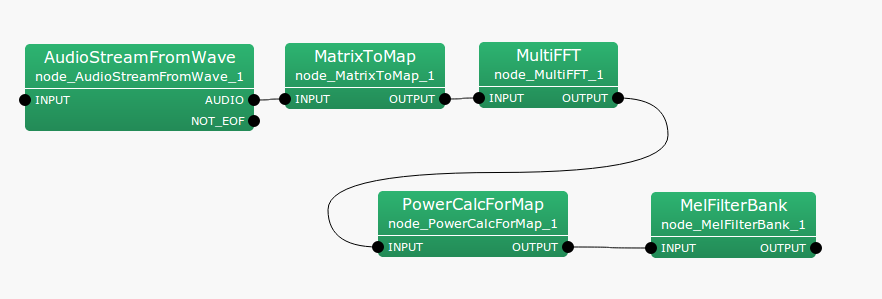## 6.7.9 PowerCalcForMap

### 6.7.9.1 Outline of the node

This node converts multichannel complex spectra of Map<int, ObjectRef> type IDs into real power/amplitude spectra.

### 6.7.9.2 Necessary files

No files are required.

### 6.7.9.3 Usage

When to use

This node is used to convert complex spectra into real power/amplitude spectra when inputs are Map<int, ObjectRef> type. When inputs are of Matrix<complex<float> > type, use the PowerCalcForMatrix node.

Typical connection

Figure 6.107 shows an example of the usage of the PowerCalcForMap node. A Map<int, ObjectRef> type complex spectrum obtained from the MultiFFT node is converted into Map<int, ObjectRef> type power spectrum and input to the MelFilterBank node.Figure 6.107: Example of a connection of PowerCalcForMap

### 6.7.9.4 Input-output and properties of the node

Table 6.79: Parameters of PowerCalcForMap
 Parameter name Type Default value Unit Description POWER_TYPE POW Selection of power/amplitude spectra

Input

INPUT

: Map<int, ObjectRef> type. Complex matrices of Matrix<complex<float> > type are stored in the ObjectRef part.

Output

OUTPUT

: Map<int, ObjectRef> type. Real matrices of power/absolute values are stored in the ObjectRef part for each element of the complex matrices of the inputs.

Parameter

POWER_TYPE

: string type. Selection of power (POW) or amplitude (MAG) spectra for the output.

### 6.7.9.5 Details of the node

The real matrix $N_{i,j}$ of the output for the complex matrix $M_{i,j}$ of an input ($i, j$ indicating the rows and columns of the index, respectively) is obtained as:

 $\displaystyle N_{i,j}$ $\displaystyle =$ $\displaystyle M_{i,j}M_{i,j}^{*}~ ~ (\textrm{if POWER\_ TYPE=POW}),$ $\displaystyle N_{i,j}$ $\displaystyle =$ $\displaystyle abs(M_{i,j})~ ~ (\textrm{if POWER\_ TYPE=MAG}),$

Here, $M_{i,j}^{*}$ indicates the complex conjugate of $M_{i,j}$.